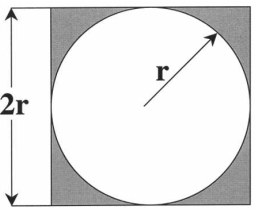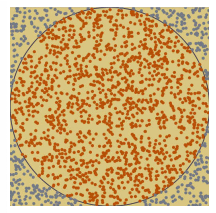## [原创] 蒙特卡罗方法的实例1：计算圆周率

• 非蒙特卡罗方法

$\frac{\pi }{4} = \sum\limits_{n = 0}^\infty {\frac{{{{\left( { - 1} \right)}^n}}}{{2n + 1}}} = 1 - \frac{1}{3} + \frac{1}{5} - \frac{1}{7} + \frac{1}{9} - \cdots$

$n$ 的最大值取30000时，可以计算得 $\pi = 3.141559320256462$

• 蒙特卡罗方法• 参考文献

➤➤ 版权声明 ➤➤